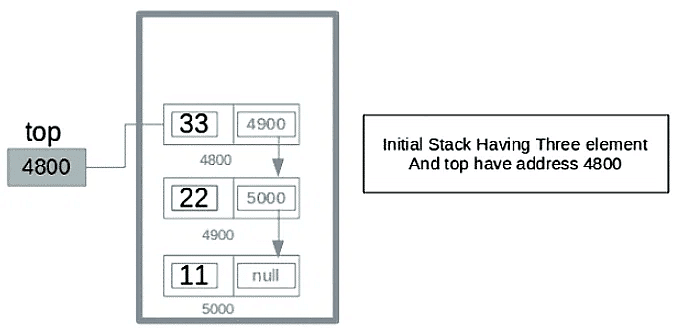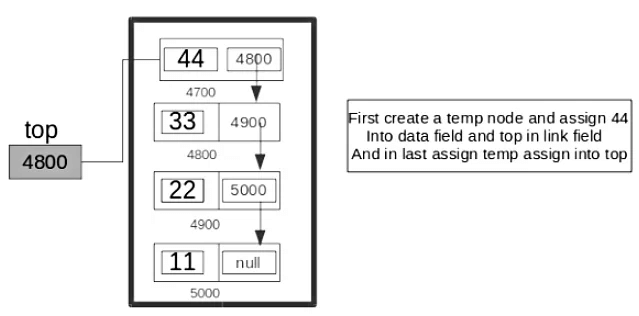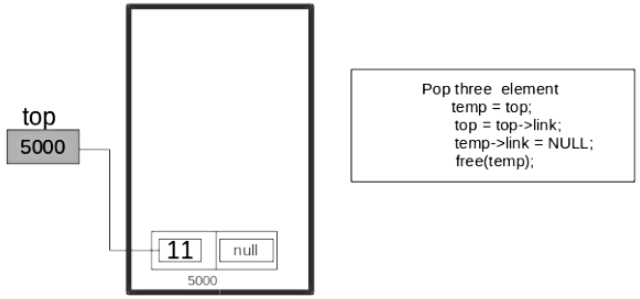# Implement Stack using Linked List - Notes | Study Programming and Data Structures - Computer Science Engineering (CSE)

 Table of contentsIntroduction1 Crore+ students have signed up on EduRev. Have you?

Introduction

To implement a stack using the singly linked list concept, all the singly linked list operations should be performed based on Stack operations LIFO(last in first out) and with the help of that knowledge, we are going to implement a stack using a singly linked list.

So we need to follow a simple rule in the implementation of a stack which is last in first out and all the operations can be performed with the help of a top variable. Let us learn how to perform Pop, Push, Peek, and Display operations in the following article:In the stack Implementation, a stack contains a top pointer. which is the “head” of the stack where pushing and popping items happens at the head of the list. The first node has a null in the link field and second node-link has the first node address in the link field and so on and the last node address is in the “top” pointer.
The main advantage of using a linked list over arrays is that it is possible to implement a stack that can shrink or grow as much as needed. Using an array will put a restriction on the maximum capacity of the array which can lead to stack overflow. Here each new node will be dynamically allocated. so overflow is not possible.

Stack Operations
• push(): Insert a new element into the stack i.e just insert a new element at the beginning of the linked list.
• pop(): Return the top element of the Stack i.e simply delete the first element from the linked list.
• peek(): Return the top element.
• display(): Print all elements in Stack.
Push Operation
• Initialise a node
• Update the value of that node by data i.e. node->data = data
• Now link this node to the top of the linked list
• And update top pointer to the current node
Pop Operation
• First Check whether there is any node present in the linked list or not, if not then return
• Otherwise make pointer let say temp to the top node and move forward the top node by 1 step
• Now free this temp node
Peek Operation
• Check if there is any node present or not, if not then return.
• Otherwise return the value of top node of the linked list
Display Operation
• Take a temp node and initialize it with top pointer
• Now start traversing temp till it encounters NULL
• Simultaneously print the value of the temp node

Below is the implementation of the above operations

C++

// C++ program to Implement a stack

#include <bits/stdc++.h>

using namespace std;

class Node {

public:

int data;

// Constructor

Node(int n)

{

this->data = n;

}

};

class Stack {

Node* top;

public:

Stack() { top = NULL; }

void push(int data)

{

// Create new node temp and allocate memory in heap

Node* temp = new Node(data);

// Check if stack (heap) is full.

// Then inserting an element would

if (!temp) {

cout << "\nStack Overflow";

exit(1);

}

// Initialize data into temp data field

temp->data = data;

// Put top pointer reference into temp link

// Make temp as top of Stack

top = temp;

}

// Utility function to check if

// the stack is empty or not

bool isEmpty()

{

// If top is NULL it means that

// there are no elements are in stack

}

int peek()

{

// If stack is not empty , return the top element

if (!isEmpty())

else

exit(1);

}

// Function to remove

// a key from given queue q

void pop()

{

Node* temp;

// Check for stack underflow

if (top == NULL) {

cout << "\nStack Underflow" << endl;

exit(1);

}

else {

// Assign top to temp

temp = top;

// Assign second node to top

// This will automatically destroy

// the link between first node and second node

// Release memory of top node

// i.e delete the node

free(temp);

}

}

// Function to print all the

// elements of the stack

void display()

{

Node* temp;

// Check for stack underflow

if (top == NULL) {

cout << "\nStack Underflow";

exit(1);

}

else {

temp = top;

while (temp != NULL) {

// Print node data

cout << temp->data;

// Assign temp link to temp

if (temp != NULL)

cout << " -> ";

}

}

}

};

// Driven Program

int main()

{

// Creating a stack

Stack s;

// Push the elements of stack

s.push(11);

s.push(22);

s.push(33);

s.push(44);

// Display stack elements

s.display();

// Print top element of stack

cout << "\nTop element is " << s.peek() << endl;

// Delete top elements of stack

s.pop();

s.pop();

// Display stack elements

s.display();

// Print top element of stack

cout << "\nTop element is " << s.peek() << endl;

return 0;

}

Output

44 -> 33 -> 22 -> 11

Top element is 44

22 -> 11

Top element is 22

Time Complexity: O(1), for all push(), pop(), and peek(), as we are not performing any kind of traversal over the list. We perform all the operations through the current pointer only.
Auxiliary Space: O(N), where N is the size of the stack

The document Implement Stack using Linked List - Notes | Study Programming and Data Structures - Computer Science Engineering (CSE) is a part of the Computer Science Engineering (CSE) Course Programming and Data Structures.
All you need of Computer Science Engineering (CSE) at this link: Computer Science Engineering (CSE)

## Programming and Data Structures

71 docs|29 tests
 Use Code STAYHOME200 and get INR 200 additional OFF

## Programming and Data Structures

71 docs|29 tests

Track your progress, build streaks, highlight & save important lessons and more!

,

,

,

,

,

,

,

,

,

,

,

,

,

,

,

,

,

,

,

,

,

;